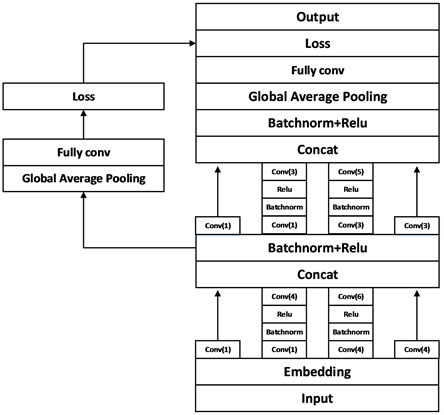# 字符级全卷积神经网络的文本分类方法Character Level Full Convolution Neural Network for Text Classification

DOI: 10.12677/CSA.2020.102024, PDF, HTML, XML, 下载: 264  浏览: 759  科研立项经费支持

Abstract: In order to solve the problem of too many parameters in the full connection layer and low calculation efficiency of the traditional convolutional neural network, the full convolutional neural network and the global average pooling layer are used in image processing for text classification, the convolutional layer is combined with the global average pooled layer and the fully connected layer is replaced. Meanwhile, using the multi-scale convolution kernel with reference to the Inception structure reduces the number of parameters, speeds up the convergence, and increases the classification accuracy of the model. In addition, in order to avoid the curse of dimensionality and the slow speed of word level vector training, character level vector representation is used. And the batch standardization layer is used instead of the Dropout layer, reducing over-fitting problems. By using multiple indicators to evaluate the model in the test data set, the validity of the model is fully verified. Compared with the traditional model, the proposed model has better classification performance in the classification task.

1. 引言

2. 相关工作

2006年Hinton  等人利用逐层贪心算法初始化深度信念网络(DBN)，提出了深层学习的概念。此后很多的深度学习模型被提出，He  等人和Abdel-Hamid  使用深度神经网络分别在计算机视觉领域和语音识别方面取得了显著的成果。

3. 模型设计与算法流程

3.1. 总模型Figure 1. Overall structure of the model

3.2. 全卷积和全局平均池化

$\text{Time}\sim \text{O}\left({\text{M}}^{\text{2}}\ast {\text{K}}^{\text{2}}\ast \text{Cin}\ast \text{Cout}\right)$ (1)

$\text{Space}\sim \text{O}\left({\text{K}}^{\text{2}}\ast \text{Cin}\ast \text{Cout}\right)$ (2)

$\text{Time}\sim \text{O}\left({\text{1}}^{\text{2}}\ast {\text{X}}^{\text{2}}\ast \text{Cin}\ast \text{Cout}\right)$ (3)

$\text{Space}\sim \text{O}\left({\text{X}}^{\text{2}}\ast \text{Cin}\ast \text{Cout}\right)$ (4)

$\text{Time}\sim \text{O}\left(\text{Cin}\ast \text{Cout}\right)$ (5)

$\text{Space}\sim \text{O}\left(\text{Cin}\ast \text{Cout}\right)$ (6)

3.3. 批量标准化

$\mu ←\frac{1}{m}\underset{i=1}{\overset{m}{\sum }}{x}_{i}$ (7)

${\sigma }_{c}^{2}←\frac{1}{m}\underset{i=1}{\overset{m}{\sum }}{\left({x}_{i}-{\mu }_{c}\right)}^{2}$ (8)

${\stackrel{^}{x}}_{i}←\frac{{x}_{i}-{\mu }_{c}}{\sqrt{{\sigma }_{c}^{2}+\epsilon }}$ (9)

${y}_{i}←\gamma {\stackrel{^}{x}}_{i}+\beta \equiv B{N}_{\gamma ,\beta \left({x}_{i}\right)}$ (10)

3.4. 字符表示

3.5. 算法实现

$\text{loss}\left(p,f\right)=-\frac{1}{n}\underset{i=1}{\overset{n}{\sum }}p\left({x}_{i}\right)\mathrm{log}\left(f\left({x}_{i}\right)\right)$ (11)

4. 实验

4.1. 超参数设置Table 1. Experimental parameters and specificationsTable 2. Convolution kernel structure

4.2. 评价标准

4.3. 实验数据

THUCnews新闻数据集：该数据集由清华大学自然语言处理实验室搜集与整理，通过过滤筛选2005~2011年间新浪新闻数据生成，包含74万篇新闻文档，均为UTF-8纯文本格式，并重新整合划分出彩票、教育、娱乐、科技等14个分类类别，所有文档均按对应类别储存在14个类别文件夹下。Table 3. Data sample distribution

4.4. 实验与结果分析

4.4.1. 对Inception结构和全卷积效果的验证Table 4. Comparison of test results of the modelFigure 2. Curve of accuracy rate with time during trainingFigure 3. Curve of loss with time during training

4.4.2. 对比实验Table 5. Comparison of accuracy between Inception-CharFcn model and other models

5. 结束语

  Hinton, G.E. and Salakhutdinov, R.R. (2006) Reducing the Dimensionality of Data with Neural Networks. Science, 313, 504-507. https://doi.org/10.1126/science.1127647  He, K., Zhang, X., Ren, S., et al. (2016) Deep Residual Learning for Image Recognition. IEEE Conference on Computer Vision and Pattern Recognition, Las Vegas, 770-778. https://doi.org/10.1109/CVPR.2016.90  Abdelhamid, O., Mohamed, A., Jiang, H., et al. (2012) Applying Convolutional Neural Networks Concepts to Hybrid NN-HMM Model for Speech Recognition. IEEE International Conference on Acoustics, Speech and Signal Processing, Kyoto, 25-30 March 2012, 4277-4280. https://doi.org/10.1109/ICASSP.2012.6288864  Kim, Y. (2014) Convolutional Neural Networks for Sentence Classification. Proceeding of the Conference on Empirical Methods in Natural Language Processing, Doha, 16. https://doi.org/10.3115/v1/D14-1181  Kim, Y., Jernite, Y., Sontag, D., et al. (2015) Character-Aware Neural Language Models. Proceedings of the Thirtieth AAAI Conference on Artificial Intelligence, Phoenix, 12-17 February 2015, 2741-2749.  Kalchbrenner, N., Grefenstette, E. and Blunsom, P. (2014) A Convolutional Neural Network for Modelling Sentences. Proceedings of the 52nd Annual Meeting of the Association for Computational Linguistics, Baltimore, 23-25 June 2014, 655-665. https://doi.org/10.3115/v1/P14-1062  Zhang, X., Zhao, J. and Lecun, Y. (2015) Character-Level Convolutional Networks for Text Classification.  Poon, H.K., Yap, W.S., Tee, Y.K., et al. (2018) Document Level Polarity Classification with Attention Gated Recurrent Unit. International Conference on Information Networking, Chiang Mai, 10-12 January 2018, 7-12. https://doi.org/10.1109/ICOIN.2018.8343074  冯兴杰, 张志伟, 史金钏. 基于卷积神经网络和注意力模型的文本情感分析[J]. 计算机应用研究, 2018(5): 1434-1436.  何炎祥, 孙松涛, 牛菲菲, 等. 用于微博情感分析的一种情感语义增强的深度学习模型[J]. 计算机学报, 2017, 40(4): 773-790.  Zhou, C., Sun, C., Liu, Z., et al. (2015) C-LSTM Neural Network for Text Classification. Computer Science, 1, 39-44.  Joulin, A., Grave, E., Bojanowski, P., et al. (2016) Bag of Tricks for Efficient Text Classification. https://doi.org/10.18653/v1/E17-2068  Lin, M., Chen, Q. and Yan, S. (2013) Network in Net-work.  Szegedy, C., Liu, W., Jia, Y., et al. (2014) Going Deeper with Convolutions. IEEE Conference on Computer Vision and Pattern Recognition, Boston, 7-12 June 2015, 1-9. https://doi.org/10.1109/CVPR.2015.7298594  Long, J., Shelhamer, E. and Darrell, T. (2014) Fully Convolutional Networks for Semantic Segmentation. IEEE Transactions on Pattern Analysis & Machine Intelligence, 39, 640-651.  张曼, 夏战国, 刘兵, 周勇. 全卷积神经网络的字符级文本分类方法[J/OL]. 计算机工程与应用, 1-11. http://kns.cnki.net/kcms/detail/11.2127.TP.20190327.1747.010.html, 2019-10-05.  Mikolov, T., Chen, K., Corrado, G., et al. (2013) Efficient Estimation of Word Representations in Vector Space.  Pennington, J., Socher, R. and Manning, C. (2014) Glove: Global Vectors for Word Representation. Conference on Empirical Methods in Natural Language Processing, Doha, 1532-1543.  Peters, M.E., Neumann, M., Iyyer, M., et al. (2018) Deep Contextualized Word Representations. Proceedings of the 2018 Conference of the North American Chapter of the Association for Computational Linguistics: Human Language Technologies, Volume 1, 2227-2237.  Ioffe, S. and Szegedy, C. (2015) Batch Normalization: Accelerating Deep Network Training by Reducing Internal Covariate Shift. International Conference on Machine Learning.